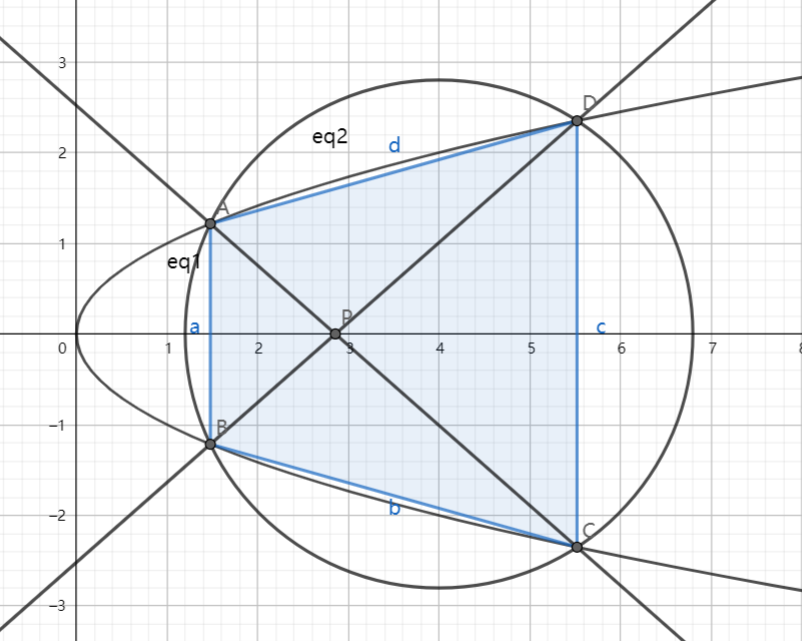# SAT1000 - P845

Geometry Level 4As shown above, the parabola $E: y^2=x$ and circle $M: (x-4)^2+y^2=r^2$ for $r>0$ intersect at points $A$, $B$, $C$, and $D$; and their relative positions are shown in the figure. Lines $AC$ and $BD$ intersect at point $P$.

Find the coordinates $(x_0,0)$ of $P$ as the area of quadrilateral $ABCD$ reaches the maximum when $r$ varies. Submit $\lfloor 1000x_0 \rfloor$.

Have a look at my problem set: SAT 1000 problems

×